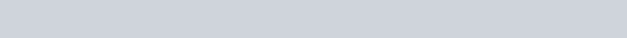Using vector method, show that the points
Question:

Using vector method, show that the points $A(4,5,1), B(0,-1,-1), C(3,9,4)$ and $D(-4,4,4)$ are coplanar.

Solution: